Einstein Equation and the Stress-Energy-Momentum Tensor

Let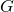denote Newton’s constant and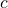denote the speed of light. The Einstein equation is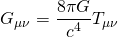where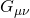is the Einstein tensor and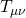is the stress-energy-momentum tensor. These equations extremize the action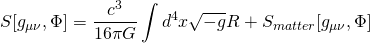where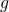is the determinant of the spacetime metric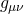and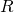is the curvature scalar. The matter fields are denoted collectively by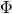. The stress-energy-momentum tensor is obtained from the matter action by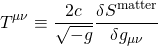Let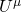denote the velocity of an observer and let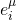denote a set of spatial, orthonormal basis vectors. We have the normalization conditions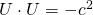,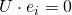, and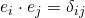. The 4-momentum density and the 4-momentum flux as seen by the observer are:

(1)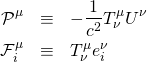From the 4-momentum density we define the energy density and 3-momentum density:

(2)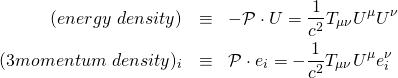From the 4-momentum flux we define the energy flux and the 3-momentum flux:

(3)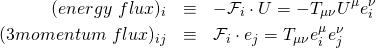Note that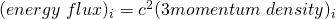. The 3-momentum flux is also identified as the spatial stress.